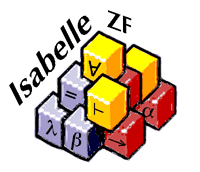Formalising Mathematics Using IsabelleGödel’s Incompleteness Theorems

Gödel’s Incompleteness Theorems are among the most celebrated (and misunderstood) results in mathematics. The first incompleteness theorem states that every sufficiently strong formal theory has an undecidable sentence; the second incompleteness theorem states that no such formal system can prove a theorem that is logically equivalent to its own consistency. While the first theorem has been mechanised using a number of proof assistants as early as 1986, the second incompleteness theorem has never been mechanised until now. The two papers below describe the work (1) for logicians and (2) for researchers in automated reasoning. The proof scripts and slides are also available.

Formalising Set Theory

Axiomatic set theory is the foundation of mathematics, but for most people it is obscure and incomprehensible. The papers described below concern Isabelle/ZF, which provides an interactive environment for undertaking set theory proofs. Isabelle's architecture, based on a logical framework, allows it to support ZF set theory within the context of first-order logic. The highlight of this work is the formalisation of Gödel's constructible universe, a feat that cannot even be attempted using most verification tools.

Miscellaneous Papers

Last revised: 8 January, 2015

Lawrence C. PaulsonComputer LaboratoryUniversity of Cambridge